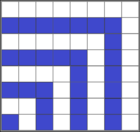## Two Important Properties of Sums (Linearity)

Summations written using sigma notation share some really nice properties with limits and derivatives (and many other important mathematical constructs), in that constant multipliers can be "pulled out", and sums and/or differences can be "pulled apart".

To be more precise: $$\sum_{i=0}^{n} c a_i = c \displaystyle{\sum_{i=0}^{n} a_i} \quad \textrm{ and } \quad \sum_{i=0}^{n} (a_i \pm b_i) = \left[ \displaystyle{\sum_{i=0}^{n} a_i} \right] \pm \left[ \displaystyle{\sum_{i=0}^{n} b_i} \right]$$ These two properties are easily proven using the distributive and commutative properties. For example, with regard to being able to "pull out" a constant multiplier, consider the following (assume $c$ represents some constant): $$\begin{array}{rcl} \displaystyle{\sum_{i=0}^{n} c a_i} &=& c a_0 + c a_1 + c a_2 + \cdots + c a_n\\ &=& c(a_0 + a_1 + a_2 + \cdots + a_n)\\ &=& c \displaystyle{\sum_{i=0}^{n} a_i}\\ \end{array}$$ Similarly, with regard to being able to "pull apart" sums and/or differences, notice $$\begin{array}{rcl} \displaystyle{\sum_{i=0}^{n} (a_i + b_i)} &=& (a_0 + b_0) + (a_1 + b_1) + (a_2 + b_2) + \cdots + (a_n + b_n)\\ &=& (a_0 + a_1 + a_2 + \cdots + a_n) + (b_0 + b_1 + b_2 + \cdots + b_n)\\ &=& \left[ \displaystyle{\sum_{i=0}^{n} a_i} \right] + \left[ \displaystyle{\sum_{i=0}^{n} b_i} \right]\\ \end{array}$$ and $$\begin{array}{rcl} \displaystyle{\sum_{i=0}^{n} (a_i - b_i)} &=& (a_0 - b_0) + (a_1 - b_1) + (a_2 - b_2) + \cdots + (a_n - b_n)\\ &=& (a_0 + a_1 + a_2 + \cdots + a_n) - (b_0 + b_1 + b_2 + \cdots + b_n)\\ &=& \left[ \displaystyle{\sum_{i=0}^{n} a_i} \right] - \left[ \displaystyle{\sum_{i=0}^{n} b_i} \right]\\ \end{array}$$

These two properties frequently allow us to express complicated sums in terms of simpler ones. For example, suppose we wish to find a formula for the sum of the first $n$ positive odd integers: $$\begin{array}{rcl} 1 + 3 + 5 + \cdots + (2n-1) &=& \displaystyle{\sum_{i=1}^{n} (2i-1)}\\ &=& \left[ \displaystyle{\sum_{i=0}^{n} 2i} \right] - \left[ \displaystyle{\sum_{i=0}^{n} 1} \right]\\ &=& \left[ 2\displaystyle{\sum_{i=0}^{n} i} \right] - \left[ \displaystyle{\sum_{i=0}^{n} 1} \right]\\ &=& \displaystyle{2 \cdot \frac{n(n+1)}{2} - n}\\ &=& (n^2 + n) - n\\ &=& n^2 \end{array}$$ So the sum of the first $n$ positive odd integers is $n^2$. Sure enough, if we check a quick example: $$1+3+5+7+9=25=5^2$$ Just for fun, note that there are other ways to show this same result -- like the following "Proof without Words":Sums of Consecutive Odd Images